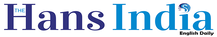Top

# No More Super-Computers To Compute Pix
Highlights

## Here is a simple but world-shaking revelation on the exact value of Pi in Mathematics. Till now we have been using Super-computers in the computation of Pi value spending many hours for many days to derive astronomical number of decimals to 3.14159265358… which is an approximate value up to its 2nd decimal only. Unknowingly, it has been believed as accurate approximate till the last decimal place of 3.14159265358… This value has been in vogue for the last 2000 years.Here is a simple but world-shaking revelation on the exact value of Pi in Mathematics. Till now we have been using Super-computers in the computation of Pi value spending many hours for many days to derive astronomical number of decimals to 3.14159265358… which is an approximate value up to its 2nd decimal only. Unknowingly, it has been believed as accurate approximate till the last decimal place of 3.14159265358… This value has been in vogue for the last 2000 years. The Holy Bible has said Pi value is 3. The first mathematician who found a simple method to compute Pi value is Eudoxus of Cnidos (500 BC) Greece. From his method, Archimedes of Syracuse (240 BC) making more scientific and has said Pi is less than 22/7 = 3.142857142857… After further refinement by later mathematicians of Archimedes’s method, the value of Pi came to be known, as 3.14159265358… which we follow even now.

However, from 1450 AD, Madhava of Kerala, a new method called infinite series was introduced from which thousands of decimals of Pi became possible. With the advent of Super Computers, Yasumasa Kanada of Department of Computer Science, Tokyo University, Japan has computed trillions of decimals of 3.14159265358… This number was also derived by many great mathematicians and are Sir Issac Newton, Leonhard Euler and S. Ramanujan, a few. It is very unfortunate their number now proved to be false.
In March 1998, the new Pi value was discovered and is (14 – root2)/4. A simple pocket calculator gives 3.14644660941… More than hundred geometrical methods confirm this value. It is an algebraic number. Whereas, the present Pi number is a transcendental number. Thank God ! Very recently a great mathematical truth is seen which gives a very simplest relation among side, diagonal of a square with circumference of its inscribed circle. The relation is : 14 sides – diagonal = 4 circumferences.
In other words 14a – (root2)a = 4 Pi a, where, side (a) is equal to the diameter (d) of its inscribed circle. From the above, Pi value can be derived very easily. A simple calculator is enough. There is no need for Super computers to compute Pi value of circle. Yet another and interesting break- through here is, the inscribed circle demarcates a length equal to diagonal from the sides of the square.
From Baudhayana (800 BC) of India and Pythagoras (450 BC) of Greece, it has been believed that a square alone can create a diagonal. But, now it is clear that an inscribed circle in a square too can demarcate a length equal to the diagonal of its superscribed square. This is yet another evidence that circle and square are one. (The writer is a retired Lecturer in Zoology) More details are available at www.rsjreddy.webnode.com.

More On
Subscribed Failed...
Subscribed Successfully...Next Story
More Stories

ADVERTISEMENTS